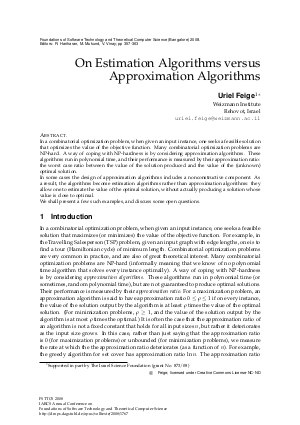Document# On Estimation Algorithms vs Approximation Algorithms

### Author Uriel Feige## File

LIPIcs.FSTTCS.2008.1767.pdf
• Filesize: 448 kB
• 7 pages

## Cite As

Uriel Feige. On Estimation Algorithms vs Approximation Algorithms. In IARCS Annual Conference on Foundations of Software Technology and Theoretical Computer Science. Leibniz International Proceedings in Informatics (LIPIcs), Volume 2, pp. 357-363, Schloss Dagstuhl - Leibniz-Zentrum für Informatik (2008)
https://doi.org/10.4230/LIPIcs.FSTTCS.2008.1767

## Abstract

In a combinatorial optimization problem, when given an input instance, one seeks a feasible solution that optimizes the value of the objective function. Many combinatorial optimization problems are NP-hard. A way of coping with NP-hardness is by considering approximation algorithms. These algorithms run in polynomial time, and their performance is measured by their approximation ratio: the worst case ratio between the value of the solution produced and the value of the (unknown) optimal solution. In some cases the design of approximation algorithms includes a nonconstructive component. As a result, the algorithms become estimation algorithms rather than approximation algorithms: they allow one to estimate the value of the optimal solution, without actually producing a solution whose value is close to optimal. We shall present a few such examples, and discuss some open questions.
##### Keywords
• Estimation Algorithms
• Approximation Algorithms
• Combinatorial Optimization

## Metrics

• Access Statistics
• Total Accesses (updated on a weekly basis)
0